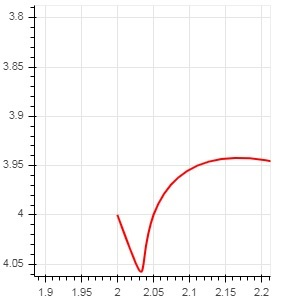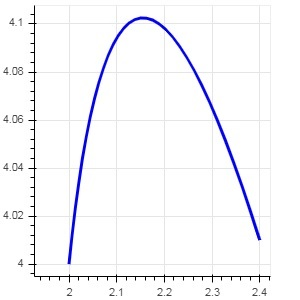# Bokeh - Specialized Curves

The bokeh.plotting API supports methods for rendering following specialised curves −

## beizer()

This method adds a Bézier curve to the figure object. A Bézier curve is a parametric curve used in computer graphics. Other uses include the design of computer fonts and animation, user interface design and for smoothing cursor trajectory.

In vector graphics, Bézier curves are used to model smooth curves that can be scaled indefinitely. A "Path" is combination of linked Bézier curves.

The beizer() method has following parameters which are defined −

 1 x0 The x-coordinates of the starting points. 2 y0 The y-coordinates of the starting points.. 3 x1 The x-coordinates of the ending points. 4 y1 The y-coordinates of the ending points. 5 cx0 The x-coordinates of first control points. 6 cy0 The y-coordinates of first control points. 7 cx1 The x-coordinates of second control points. 8 cy1 The y-coordinates of second control points.

Default value for all parameters is None.

### Example

Following code generates a HTML page showing a Bézier curve and parabola in Bokeh plot −

```x = 2
y = 4
xp02 = x+0.4
xp01 = x+0.1
xm01 = x-0.1
yp01 = y+0.2
ym01 = y-0.2
fig = figure(plot_width = 300, plot_height = 300)
fig.bezier(x0 = x, y0 = y, x1 = xp02, y1 = y, cx0 = xp01, cy0 = yp01,
cx1 = xm01, cy1 = ym01, line_color = "red", line_width = 2)
```

### OutputThis method adds a parabola glyph to bokeh figure. The function has same parameters as beizer(), except cx0 and cx1.

### Example

The code given below generates a quadratic curve.

```x = 2
y = 4
xp02 = x + 0.3
xp01 = x + 0.2
xm01 = x - 0.4
yp01 = y + 0.1
ym01 = y - 0.2
x = x,
y = y,
xp02 = x + 0.4,
xp01 = x + 0.1,
yp01 = y + 0.2,
fig.quadratic(x0 = x, y0 = y, x1 = x + 0.4, y1 = y + 0.01, cx = x + 0.1,
cy = y + 0.2, line_color = "blue", line_width = 3)
```

### Output